Next: Landau Parameters from the Up: Gamow-Teller strength and the Previous: Pressure, Incompressibility and Asymmetry

# Landau Parameters from the Skyrme energy functional

A simple and instructive description of the residual interaction in homogeneous INM is given by the Landau interaction developed in the context of Fermi-liquid theory . Landau parameters corresponding to the Skyrme forces are discussed in Refs. [21,27,36,37,38,73]. Starting from the full density matrix in (relative) momentum space
, the various densities are defined as
=
(55)
=
(56)
=
(57)
=
(58)

The kinetic densities are given by
,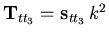. The Landau-Migdal interaction is defined as
=
=
(59)

The isoscalar-scalar, isovector-scalar, isoscalar-vector, and isovector-vector channels of the residual interaction are given by
=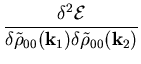(60)
=
(61)
=
(62)
=
(63)

Assuming that only states at the Fermi surface contribute, i.e.,
,
,
,
, and
depend on the angle
between
andonly, and can be expanded into Legendre polynomials, e.g.
(64)

The normalization factor N0 is the level density at the Fermi surface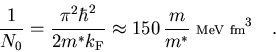(65)

A variety of definitions of the normalization factor N0 are used in the literature and great care has to be taken when comparing values from different groups; see, e.g., Ref. for a detailed discussion. We use the convention defined in . The Landau parameters corresponding to the general energy functional (6) are
 f0 =
f0' =
g0 =
g0 =
f1 =
f1' =
g1 =
g1' =
(66)

Higher-order Landau parameters vanish for the second-order energy functional (12), but not for finite-range interactions as the Gogny force discussed in the next Appendix. The Landau parameters provide a stability criterion for symmetric unpolarized INM: It becomes unstable for a given interaction when either
,
,
, or
is less than
.Next: Landau Parameters from the Up: Gamow-Teller strength and the Previous: Pressure, Incompressibility and Asymmetry
Jacek Dobaczewski
2002-03-15